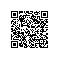# MySQL查看数据库、表的占用空间大小以及某个库中所有表的引擎类型

 代码如下 复制代码 SELECT SUM(DATA_LENGTH)+SUM(INDEX_LENGTH) FROM information_schema.tables WHERE TABLE_SCHEMA='database_name';

 代码如下 复制代码 SELECT TABLE_NAME,DATA_LENGTH+INDEX_LENGTH,TABLE_ROWS FROM information_schema.tables WHERE TABLE_SCHEMA='database_name' AND TABLE_NAME='table_name';

 代码如下 复制代码 sELECT TABLE_NAME,UPDATE_TIME FROM information_schema.tables where TABLE_SCHEMA='database_name';  LOG

information_schema 库的 TABLES 表，主要字段分别是：

TABLE_SCHEMA : 数据库名
TABLE_NAME：表名
ENGINE：所使用的存储引擎
TABLES_ROWS：记录数
DATA_LENGTH：数据大小
INDEX_LENGTH：索引大小

 代码如下 复制代码 //先进去MySQL自带管理库：information_schema  //自己的数据库：dbwww58com_kuchecarlib  //自己的表：t_carmodelparamvalue mysql> use information_schema; Database changed mysql> select data_length,index_length     -> from tables where     -> table_schema='dbwww58com_kuchecarlib'     -> and table_name = 't_carmodelparamvalue'; +-------------+--------------+ | data_length | index_length | +-------------+--------------+ |   166379520 |    235782144 | +-------------+--------------+ 1 row in set (0.02 sec) mysql> select concat(round(sum(data_length/1024/1024),2),'MB') as data_length_MB,     -> concat(round(sum(index_length/1024/1024),2),'MB') as index_length_MB     -> from tables where     -> table_schema='dbwww58com_kuchecarlib'     -> and table_name = 't_carmodelparamvalue'; +----------------+-----------------+ | data_length_MB | index_length_MB | +----------------+-----------------+ | 158.67MB       | 224.86MB        | +----------------+-----------------+ 1 row in set (0.03 sec)

for i in $(cat tables.txt); do mysql --user=*** --password=*** -h 192.168.*.* 库名 -e "show create table$i;"  | awk -F"ENGINE" '{print $2}' | awk '{print$1}' ;done  | grep -v ^$;done for循环使用示例：for i in seq 3;do echo$i; done

本文转自Tenderrain 51CTO博客，原文链接：http://blog.51cto.com/tenderrain/1610463，如需转载请自行联系原作者使用钉钉扫一扫加入圈子
+ 订阅